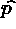Next: About this document Up: My Home Page

# Linked Stacks and Queues Lecture 5

Steven S. Skiena

Pointers about Pointers

`var p, q : ^node;`

p = new(node) creates a new node and sets p to point to it.

pdescribes the node which is pointed to by p.

p.item describes the item field of the node pointed to by p.

dispose(p) returns to the system the memory used by the node pointed to by p. This is not used because of Modula-3 garbage collection.

NIL is the only value a pointer can have which is not an address.

Linked Stacks

The problem with array-based stacks are that the size must be determined at compile time. Instead, let's use a linked list, with the stack pointer pointing to the top element.

To push a new element on the stack, we must do:

```p^.next = top;
top = p;```

Note this works even for the first push if top is initialized to NIL!

Popping from a Linked Stack

To pop an item from a linked stack, we just have to reverse the operation.

```p = top;
top = top^.next;
p^.next = NIL;    (*avoid dangling reference*)```

Note again that this works in the boundary case of one item on the stack.

Note that to check we don't pop from an empty stack, we must test whether top = NIL before using top as a pointer. Otherwise things crash or segmentation fault.

Linked Stack in Modula-3

```MODULE Stacks;               (*14.07.94 RM, LB*)
(* Implementation of the abstract, generic stack. *)
REVEAL
T = BRANDED REF RECORD
info: ET; next: T;
END; (*T*)

PROCEDURE Create(): T = (*creates and intializes a new stack*)
BEGIN
RETURN NIL;             (* a new, empty stack is simply NIL *)
END Create;

PROCEDURE Push(VAR stack: T; elem:ET) =
(*adds element to stack*)
VAR new: T := NEW(T, info:= elem, next:= stack);  (*create element*)
BEGIN
stack:= new                  (*add element at top*)
END Push;

PROCEDURE Pop(VAR stack: T): ET =
(*removes and returns top element, or NIL for empty stack*)
VAR first: ET := NIL;          (* Pop returns NIL for empty stack*)
BEGIN
IF stack # NIL THEN
first:= stack.info;        (*copy info from first element*)
stack:= stack.next;        (*remove first element*)
END; (*IF stack # NIL*)
RETURN first;
END Pop;

PROCEDURE Empty(stack: T): BOOLEAN =
(*returns TRUE for empty stack*)
BEGIN
RETURN stack = NIL
END Empty;

BEGIN
END Stacks.```

Generic Stack Interface

```INTERFACE Stacks;  (*14.07.94 RM, LB*)
(* Abstract generic stack. *)

TYPE
T <: REFANY;  (*type of stack*)
ET = REFANY;  (*type of elements*)

PROCEDURE Create(): T;                  (*creates and intializes a new stack*)

PROCEDURE Push(VAR stack: T; elem: ET); (*adds element to stack*)
PROCEDURE Pop(VAR stack: T): ET;        (*removes and returns top element, or
NIL for empty stack*)
PROCEDURE Empty(stack: T): BOOLEAN;     (*returns TRUE for empty stack*)

END Stacks.```

Generic Stacks Client

```MODULE StacksClient EXPORTS Main; (* LB *)
(* Example client of both the generic stack and the type FractionType.
This program builds up a stack of fraction numbers as well as of
complex numbers.
*)

IMPORT Stacks;
IMPORT FractionType;
FROM Stacks IMPORT Push, Pop, Empty;
FROM SIO IMPORT PutInt, PutText, Nl, PutReal, PutChar;

TYPE
Complex = REF RECORD r, i: REAL END;

VAR
stackFraction: Stacks.T:= Stacks.Create();
stackComplex : Stacks.T:= Stacks.Create();

c: Complex;
f: FractionType.T;

BEGIN (*StacksClient*)
PutText("Stacks Client\n");

FOR i:= 1 TO 4 DO
Push(stackFraction, FractionType.Create(1, i));  (*stores numbers 1/i*)
END;

FOR i:= 1 TO 4 DO
Push(stackComplex, NEW(Complex, r:= FLOAT(i), i:= 1.5 * FLOAT(i)));
END;

WHILE NOT Empty(stackFraction) DO
f:= Pop(stackFraction);
PutInt(FractionType.Numerator(f));
PutText("/");
PutInt(FractionType.Denominator(f), 1);
END;
Nl();

WHILE NOT Empty(stackComplex) DO
c:= Pop(stackComplex);
PutReal(c.r);
PutChar(':');
PutReal(c.i);
PutText("  ");
END;
Nl();

END StacksClient.```

Linked Queues

Queues in arrays were ugly because we need wrap around for circular queues. Linked lists make it easier.

We need two pointers to represent our queue - one to the rear for enqueue operations, and one to the front for dequeue operations.

Note that because both operations move forward through the list, no back pointers are necessary!

Enqueue and Dequeue

To enqueue an item:

```p^.next := NIL;
if (back = NIL) then begin    (* empty queue *)
front := p;  back := p;
end else begin                (* non-empty queue *)
back^.next := p;
back := p;
end;```

To dequeue an item:

```p := front;
front := front^.next;
p^.next := NIL;
if (front = NIL) then back := NIL;   (* now-empty queue *)```Next: About this document Up: My Home Page

Steve Skiena
Tue Sep 16 13:55:02 EDT 1997